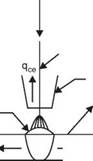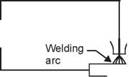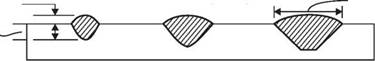WELDING PARAMETERS AND THEIR EFFECTS

Weld quality, and weld deposition rate both are influenced by various welding parameters and joint geometry. These parameters are the process variables as given below :

1. Welding current

2. Arc Voltage

3. Welding speed.

4. Electrode Feed rate

5. Electrode extension (stick-out)

6. Electrode diameter

7. Joint geometry.

Each of the above parameters affects, to varying extent, the following:

1. Deposition rate

3. Depth of penetration

4. Cooling rate

5. Weld induced distortion.

Hence, a proper understanding of the effects of welding parameters (or process vari­ables is important to obtain a sound welded joint with adequate metal deposition rate and minimum distortion. General effect of these variables will be discussed in the following para­graphs.

3.9.1 Welding Current

Melting rate is directly proportional to the energy (current and voltage) used for a given elec­trode and polarity used in DC welding. Part of this energy Q is used to melt the base metal (qb), part goes to melt electrode and flux (qf) rest is dissipated as conduction (qep + qce), convection (qv) and radiation (qr)

Q=qb + qf + (qCp + qJ + qv + q)

Also, Q = IV. J/S

= I2 Ra J/S

where Q = electrical energy consumed

I = welding current

V = arc voltage

Ra = arc resistance

 Q

 Conduction to electrode

 (used for melting qf electrode + flux)

 Electrode

 qcp conduction to plate

 cpq

Fig. 3.22 Heat balance in SAW

Welding current is most important variable affecting melting rate, the deposition rate, the depth of penetration and the amount of base metal melted.

If the current (for a given welding speed) is too high, it will result in:

• excessive penetration (thinner plates will melt through)

• Excessive melting of electrode—excessive reinforcement

• More heat input to plates being joined increased distortions If the welding current is too low, it will result in:

• lack of fusion

Current could be DC or AC. DC provides steady arc and smooth metal transfer, good wetting action, uniform weld bead size, specially suited to thin section welding, give better quality welds in vertical and overhead welding positions.

3.9.2 Arc Voltage

Arc voltage is the voltage between the job and the electrode during welding. For a given elec­trode it depends upon the arc length. Open circuit voltage on the other hand is the voltage generated by the power source when no welding is done.

Open circuit voltage varies between 50-100 V whereas arc-voltages are between 17 V to 40 V. When the arc is struck, the open circuit voltage drops to arc voltage and welding load comes on power supply.

The arc voltage depends on arc length and type of electrode.

As arc length increases, arc resistance increases, (resulting in higher voltage drop (i. e., arc-voltage increases and arc current decreases. This decrease in current depends upon the slope of volt-ampere curve explained earlier.

Arc length is the distance between the molten electrode tip to the surface of molten weld pool. Proper arc length is important in obtaining a sound joint. As the metal droplet transfers through the arc there is a variation in instantaneous arc voltage. Welding will be quite smooth if the arc voltage variation and hence the arc length is maintained consistant. As a general rule arc length should not be more than the electrode diameter.

 o® @
 Welding її І torch v(>)
 PlateArc- voltage

Power —v source T'TN

Open circuit voltage Fig. 3.23 Concept of open circuit voltage and arc-voltage

 Weld reinforcement

 Depth of penetration

 2.4 mm wire, 500 A, 10 mm/s

 25 V

 35 V

 45 V

 Fig. 3.24 Effect of arc-voltage variations on weld bead shapeWeld width

Short arc: causes short circuits during metal transfer

Long arc—lacks direction and intensity, gives heavy spatter, low deposition rate and formation of undercuts.

Though arc length needs to be controlled in order to obtain a quality welding, it is much easier to monitor and control arc voltage.

Weld-bead appearance depends on arc-voltage. Increase in arc-voltage tends to cause porosity, spatter flatten the weld bead and increase weld width. Reduction in arc-voltage leads

to : narrower weld-bead, higher crown, deeper penetration. Trials are, therefore, made to obtain optimum arc voltage.